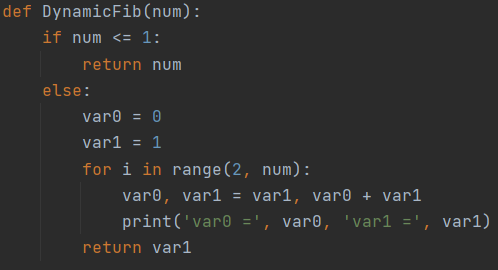# Calculating Fibonacci Series using Dynamic Programming

Fib(n) = Fib(n-1) + Fib(n-2) if n >= 2

Var0 = 0

Var1 = 1

Set Var0 to Var1 and Var1 to Var0 + Var1

Var0 = 1

Var1 = 1

F3 = Var1 = 1

Var0 = Var1 = 1

Var1 = Var1 + Var0 = 1 + 1 = 2

F4 = 2(Note: Above code was implemented using Python and a semi-competent developer)

--

--